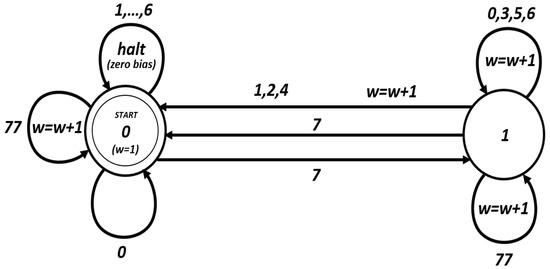Next Article in Journal
New Cryptanalytic Attack on RSA Modulus N = pq Using Small Prime Difference Method
Previous Article in Journal
CMCC: Misuse Resistant Authenticated Encryption with Minimal Ciphertext Expansion

Metrics 0

## Export Article

Open AccessArticle
Cryptography 2019, 3(1), 1; https://doi.org/10.3390/cryptography3010001

# Further Observations on SIMON and SPECK Block Cipher Families

Faculty of Mathematical and Computer Sciences, Kharazmi University, Tehran 19678, Iran
Received: 7 November 2018 / Revised: 15 December 2018 / Accepted: 18 December 2018 / Published: 20 December 2018
|   PDF [359 KB, uploaded 20 December 2018]   |

# Abstract

SIMON and SPECK families of block ciphers are well-known lightweight ciphers designed by the NSA. In this note, based on the previous investigations on SIMON, a closed formula for the squared correlations and differential probabilities of the mapping $ϕ ( x ) = x ⊙ S 1 ( x )$ on $F 2 n$ is given. From the aspects of linear and differential cryptanalysis, this mapping is equivalent to the core quadratic mapping of SIMON via rearrangement of coordinates and EA -equivalence. Based on the proposed explicit formula, a full description of DDT and LAT of $ϕ$ is provided. In the case of SPECK, as the only nonlinear operation in this family of ciphers is addition mod $2 n$ , after reformulating the formula for linear and differential probabilities of addition mod $2 n$ , straightforward algorithms for finding the output masks with maximum squared correlation, given the input masks, as well as the output differences with maximum differential probability, given the input differences, are presented. By the aid of the tools given in this paper, the process of the search for linear and differential characteristics of SIMON and SPECK families of block ciphers could be sped up, and the complexity of linear and differential attacks against these ciphers could be reduced. View Full-Text
FiguresFigure 1

This is an open access article distributed under the Creative Commons Attribution License which permits unrestricted use, distribution, and reproduction in any medium, provided the original work is properly cited (CC BY 4.0).MDPI and ACS Style

Dehnavi, S.M. Further Observations on SIMON and SPECK Block Cipher Families. Cryptography 2019, 3, 1.

Note that from the first issue of 2016, MDPI journals use article numbers instead of page numbers. See further details here.

1